# Deploying a basic Streamlit app to Heroku

## This article demonstrates the deployment of a basic Streamlit app (that simulates the Central Limit Theorem) to Heroku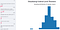Image by author

Streamlit is an app framework to deploy machine learning apps built using Python. It is an open-source framework which is similar to the Shiny package in R. Heroku is a platform-as-a-service (PaaS) that enables deployment and managing applications built in several programming languages in the cloud.

According to Central Limit Theorem, as the sample size increases, closer would be the mean of the sample means to the population mean. The distribution of the sample means (a.k.a. sampling distribution of sample means) also looks more Gaussian, irrespective of the underlying population distribution, as the sample size increases, given a sufficient number…

# Deploying a basic Streamlit app

## This article demonstrates the deployment of a basic Streamlit app (that predicts the Iris’ species) to Streamlit Sharing.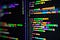Photo by Joan Gamell on Unsplash

Streamlit is an app framework to deploy machine learning apps built using Python. It is an open-source framework which is similar to the Shiny package in R. This article assumes the reader to have basic working knowledge of Conda environment, Git and machine learning with Python.

# Model Development

## Model 1

We’ll fit a Logistic Regression model to the Iris dataset from the Scikit-Learn package. The code below splits the dataset into train and test sets, to evaluate the model after deployment on the test set. We’ll use the mutual information metric for feature selection using ‘SelectKBest’ method. …

# Beginner’s guide to SQL for Data Analysis using PostgreSQL

## This article discusses a few basic SQL commands useful for data analysis. This article doesn’t cover advanced techniques involving multiple tables.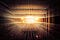Photo by Joshua Sortino on Unsplash

# Structured Query Language (SQL)

SQL is a language used to mange relational databases, where data is stored in the form of tables. A table in a relational database management system (RDBMS) is similar to a spreadsheet where each column is called a field and each row is called a record. ‘name’, ‘age’, ‘gender’ are few examples of fields.

# Data Import

We’ll use the Titanic dataset from Kaggle and import it into Postgres/PostgreSQL. Below is the process to import the data into Postgres.

Step 1:

Right click on ‘Databases’ and select ‘Create’ -> ‘Database’ and type the name of the database and click ‘Save’.

# The right way of using SMOTE with Cross-validation

## This article discusses the right way to use SMOTE to avoid inaccurate evaluation metrics while using cross-validation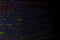Image by Mitchell Luo on Unsplash

This article assumes the reader to have a working knowledge of SMOTE, an oversampling technique to handle imbalanced class problem. We’ll discuss the right way to use SMOTE to avoid inaccurate evaluation metrics while using cross-validation techniques. First, we’ll look at the method which may result in an inaccurate cross-validation metric. We’ll use the breast cancer dataset from Scikit-Learn whose classes are slightly imbalanced.

# Understanding the scope of the variables in Python

## This article discusses the scope of the variables in Python, which is one of the fundamental concepts of Python programming.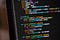Image by Chris Ried on Unsplash

Scope of a variable is the region in the code where the variable is available/accessible. A variable declared outside a function (i.e. the main region of the code) is called a global variable and a variable declared inside a function is called a local variable of that function.

`################## GLOBAL VARIABLES##################def x:    ################     LOCAL VARIABLE    ################`

Let’s look at an example to understand it better. In the below example, we declare a variable named ‘global_variable’ in the main section of the code and a variable named ‘local_variable’ inside a function.

`global_variable = 1def function(): local_variable = 2…`

# Understanding List Comprehensions in Python

## This article discusses list comprehensions in Python and how to use them to make your code more efficient and Pythonic.Image by Chris Ried on Unsplash

List comprehensions help you in performing basic list operations with minimal code (usually with a single line of code). This makes your code efficient and Pythonic. Let’s look at an example to make the concept of list comprehensions clearer.

Let’s create a list of integers from 0 to 9 and multiply each of the element in the list by 2. This can be done by iterating through each of the elements in the list using a for loop and multiply it by 2 and append it to an empty list.

`x = list(range(10))x`

# Creating Custom Transformers with Scikit-Learn

## This article discusses two methods to create custom transformers with Scikit-Learn and their implementation with Pipeline and GridSearchCV.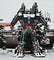Photo by Arseny Togulev on Unsplash

Transformers are classes that enable data transformations while preprocessing the data for machine learning. Examples of transformers in Scikit-Learn are SimpleImputer, MinMaxScaler, OrdinalEncoder, PowerTransformer, to name a few. At times, we may require to perform data transformations that are not predefined in popular Python packages. In such cases, custom transformers come to the rescue. In this article, we’ll discuss two methods of defining custom transformers in Python using Scikit-Learn. We’ll use the ‘Iris dataset’ from Scikit-Learn and define a custom transformer for outlier removal using the IQR method.

## Method 1

This method defines a custom transformer by inheriting BaseEstimator and TransformerMixin classes…

# How to prevent Data Leakage while evaluating the performance of a Machine Learning model

## This article discusses the problem of data leakage while evaluating a model’ performance and the ways to avoid it.Image by Chris Ried on Unsplash

Data leakage during model evaluation occurs when data from the training set passes into the validation/test set. This causes the model’ performance estimate on the validation/test set to be biased. Let’s understand it with an example using the ‘Boston house prices’ dataset from Scikit-Learn. The dataset has no missing values, hence, a hundred missing values are introduced randomly for better demonstration of data leakage.

# Mining the ‘2020 Kaggle Machine Learning & Data Science Survey’ data

This article applies Apriori algorithm to the ‘2020 Kaggle Machine Learning & Data Science Survey’ data to find out the associations among the technologies used by the respondents. This article assumes the reader to have a working knowledge of Apriori algorithm and its implementation in Python.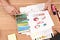Photo by William Iven on Unsplash

2020 Kaggle Machine Learning & Data Science Survey was a survey conducted by Kaggle in 2020. The survey was conducted in October 2020 online. After data curation, the survey had 20,036 responses. …

# How to decide on the number of bins of a Histogram?

## This article explains two commonly used methods to calculate the number of bins of a histogram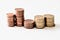Image by Ibrahim Rifath on Unsplash

## What is a histogram?

A histogram plots the frequency (count) of a numeric variable by splitting it into bins (intervals). The x-axis of a histogram has the bins and the y-axis has the frequency of samples in those bins. Shape of a histogram may vary by the number of bins. Hence, it is important to choose the right number of bins to correctly view the distribution of a numeric variable. Below is an example to show the varying shape of a histogram with the number of bins.## KSV Muralidhar

I use Python, R, SQL & Excel for data analysis, ML, web scraping & process automation as a part of my job. I also actively work on Kaggle datasets.